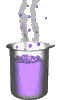Science class online
Chemistry and physics lessons

# Electricity

Free online electricity lessons for elementary school,  middle school and high school.Science class Chemistry Electricity Optics Mechanics Electricity lessons Electrical components -Two-terminal electronic components - Basic electrical components - Diodes - What's a resistor ? - How to determine a resistor value ? - Resistors effects in circuits - How to use a resistor ? - Characteristic curve of a resistor How to build simple circuits and draw diagrams - How to build a basic electrical circuit - How to draw diagrams of electric circuits The electric current - Conductors and insulators - Direction of electric current in a circuit - The dangers of electricity - Current intensity - units how to measure current ? The Voltage - The voltage and its units - How to measure a voltage ? - Voltage in open and closed circuits - Rated current and voltage for a lamp Alternating voltage and current - Alternating currents effects on led - What are alternating current and voltage ? - Periodic alternating voltage and its properties - Oscilloscope - Oscillogram - Frequency - Measuring RMS voltage with a voltmeter Serie circuits - What's a serie circuit ? - Series circuit properties - Short circuit in  series circuit - Current law in series - circuits - Voltage law in series circuits Parallel circuits - What's a parallel circuit ? - Some parallel circuits properties - Short circuits in parallel circuits - Nodes and branches in parallel circuits - Current laws in parallel circuits - Voltage laws in parallel circuits Laws of electricity - Ohm's law - Current laws in series circuits - Voltage laws in series circuits - Current laws in parallel circuits - Voltage laws in parallel circuits Generating electricity - Voltage for coil wires What is an alternator ? - Alternating currents and voltages - Generating electricity in power plants Electric power and energy - Electric power and power rating - Electric power consumption by an electrical device - Relationship between - Electric power and energy

 _____________________________________________________   _____________________________________________________

## Series circuits

### Series circuit properties

 1) Order of electric components In a series circuit if we permute two different bulbs then each bulb maintains its brightness. More generally: In a series circuit, modifying the order of connection does not affect the way electric components operate. 2) Number of passive components If we add a second bulb in a circuit including a bulb and a battery then the brightness decreases. The brightness decreases even more if we add a third. The result would be the same by adding a motor or a resistor instead of a bulb. The more a series circuit includes passive components,  the more its bulbs get brighter. ______________________________________ ______________________________________ 3) Burnt out bulb or unscrewed bulb If a bulb is burnt out or is unscrewed in a series circuit including multiple bulbs then all bulbs stop to shine. If a bulb burns out then the filament is broken and when a lamp is unscrewed there is no more contact between the bulb and the socket. In both cases the circuit is open and the electric current can not flow. Conclusion: In a series circuit if a bulb is burned out or unscrewed other passive components do not work anymore. ______________________________________ ______________________________________
____________________________________

____________________________________